The concept of valency is simple, and rationalises the atomic composition of a large number of compounds. Yet, in many chemistry courses, it is sidelined in favour of electronic theories of bonding, which are more difficult

In Short
• Modern treatment of valency, showing how simple and effective it is
• If students know the formulae of a few well-known compounds - H2O, COetc - they can work out most of the important valencies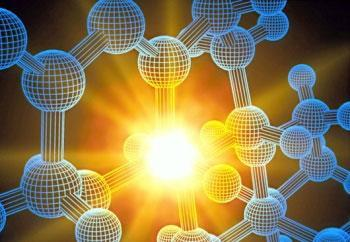Source: Thomas Hollyman/SPL

Chemical bonding

When the theory of valency was devised, chemists thought that all compounds were molecular. We now know that many are non-molecular, ie they comprise a large number of atoms bound together in a continuous framework.1 The theory can, however, be adapted to include non-molecular compounds. In this article I shall distinguish the two types by writing the formulae of non-molecular compounds as exemplified by (NaCl)∞.

### Definition

Valency can be defined as the capacity of an atom or radical to combine with other atoms or radicals. There are several different kinds.2 The simplest and most general is the 'principal', 'primary', or 'classical' valency, v. This is equal to the number of hydrogen or fluorine atoms (Y) with which an atom or radical X will combine to form a unit (XYv) of a compound. The unit can be a molecule or the formula unit of a non-molecular compound (XYv)∞. It can also be the unit of an oligomer (XYv)n. The compound must not be metallic or semi-metallic because valency does not generally hold for such compounds.3 There must also be no X-X bonds, or X atoms bound in more than one way.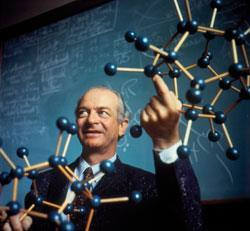Source: Pasieka/Science Photo Library

Linus Pauling - his early research focused on the nature of the chemical bond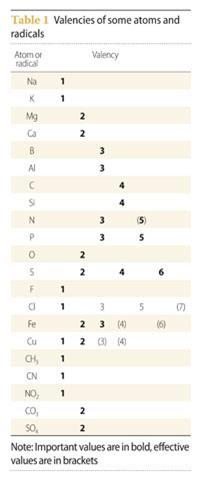Some atoms, while not forming compounds comprising XYv units, form derivatives of these. For example, nitrogen atoms do not form NH5 or NF5, but they do form derivatives of the latter in which pairs of fluorine atoms are replaced by bivalent oxygen atoms (F3NO, FNO2, and N2O5 gas). Similarly, copper atoms do not form (CuH3)∞ or (CuF3)∞, but they do form complex compounds of the fluoride with alkali metal fluorides, eg (K3[CuF6])∞. Such atoms have an 'effective' valency of v

Principal valencies of selected atoms and radicals are given in Table 1. Effective values are in brackets. I have excluded values that apply only to one species (eg CO, NO, NO2, and ClO2).

### Application

The atomic composition of compounds can be predicted by applying the principle that, in a compound the valencies of all the atoms or radicals in it are satisfied. This can be done by supposing that atoms and radicals form 'bonds' with each other, and that the number of bonds formed between two atoms or radicals is equal to the number of valencies each satisfies on the other. Thus, for example, in the CO2 molecule, there are two bonds between the carbon atom and each oxygen atom, the valency of the latter satisfying, and being satisfied by, two of the valencies of the former. This gives the familiar formula:

O=C=O

The number of bonds between two atoms or radicals is called 'bond number' and is given the symbol n.

### Integral bonds

Three cases can now be distinguished. The simplest is where all the bonds are integral (n = 1, 2, 3.). In this case, valency can be represented by short strokes (eg H-, -S-) and the satisfaction of valency by matching up strokes (eg H- -S- -S - -H). This leads to formulae of the kind exemplified for CO2

A complication is that, in general, the theory gives several possible structures for a compound. For example, a dioxide can have any of the following structures (with any angles):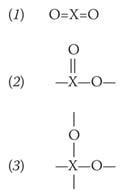Under ordinary conditions, carbon dioxide and sulfur dioxide have structure (1), selenium dioxide has structure (2), and silicon dioxide structure (3). Which structure an atom adopts depends on its size, but also on other factors.

### Non-integral bonds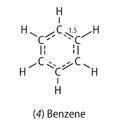At one time chemists thought only in terms of integral bonds. As a result, they were unable to draw satisfactory formulae for molecules like benzene. They have since come to realise that bonds can be non-integral. This enables a simple formula to be written for benzene (4), with equivalent bonds in the ring:

In this formula, there are one and a half bonds between the carbon atoms. This gives a total of four bonds round each carbon atom. The bond number is consistent with the fact that the carbon-carbon bond length (1.397 Å) is intermediate between that of a single bond (1.536 in ethane) and a double bond (1.339 Å in C2H4).

A second example is provided by a layer of graphite. A formula for this can be drawn with one and a third bonds between the carbon atoms. The bond length (1.421 Å) is again consistent with this, lying between that in ethane and benzene.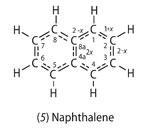A third example is the naphthalene molecule (5). A formula for this can be written as: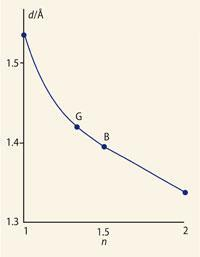Plot of bond length (d) against bond number (n) for C-C to C=C bonds

B, benzene; G, graphite

This formula is derived by setting the bond number of the 1-2 bond equal to 1 + x, and working out the bond numbers of the other ring bonds by requiring that the total number of bonds to each carbon atom should equal four. (Start with atom 2 to get the number for 2-3, then consider atom 1 to get the number for 1-8a, and finally atom 8a to get the number for 4a-8a.) A value for x can be obtained by plotting bond length against bond number for known bond numbers (Fig 1, right) and using this graph to obtain bond numbers in naphthalene (Table 2). The average value of is about 2/3.

Formulae of this type can be deduced by first assuming that all the bonds are integral. If this leads to two or more equivalent formulae, like the Kekulé structures for benzene, these can then be reduced to a single formula by using non-integral bonds.

### Fractional bonds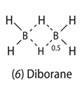Non-integral bonds can be fractional (n < 1). In the diborane molecule (6), for example, the bridging bonds are half bonds:

This gives a total of three bonds round each boron atom, and one round each hydrogen atom. The bridge bonds are longer than the terminal ones (1.32 Å compared with 1.20 Å).

Fractional bonds are common in compounds between metals and non-metals. These generally have framework structures, with each metal atom surrounded by several non-metal atoms and vice versa. An example is aluminium nitride, (AlN)∞. This has the wurtzite structure. In this, each aluminium atom is surrounded by four nitrogen atoms and each nitrogen atom by four aluminium atoms. The triple valency of each aluminium atom is therefore shared with four nitrogen atoms, so there is only three quarters of a bond between each aluminium atom and nitrogen atom (Table 3).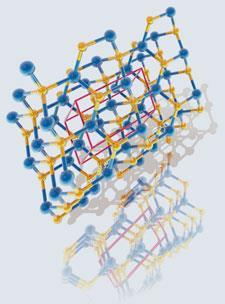The wurtzite crystal structure

I have resisted here referring to ions. Along the isoelectronic series (SiC)∞, (AlN)∞, (MgO)∞, and (NaF)∞, the bonding doubtless becomes more ionic, but we do not know how rapidly. The simple theory I am describing does not depend on bond type, and holds as well at the ionic limit as at the covalent.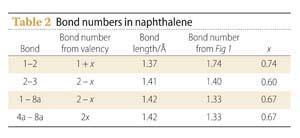The atomic composition of compounds of this kind can be predicted by supposing that bonds are integral. For aluminium nitride, this gives Al≡N. The actual bond number depends on the structure.

There are several applications of fractional bond numbers.5 One is to use the numbers in simple compounds to predict the structures of more complicated ones. For example, the bond numbers in magnesium oxide and silicon dioxide are respectively 1/3 and 1 (Table 3). If these are taken as typical of magnesium-oxygen and silicon-oxygen bonds, we can draw a structure for magnesium silicate in which each oxygen atom is bound to one silicon atom and three magnesium atoms. This gives us the structure of olivine, (Mg2SiO4)∞.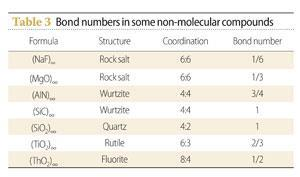Another application is as an aid in determining the structures of silicate minerals. In these, there is generally a good correlation between fractional bond number and bond length (cf Fig 1). This correlation can be used to determine bond numbers in a proposed structure, to see whether they add up to the expected valencies.

### Electrovalency

Studies show that strong electrolytes in dilute aqueous solution consist of charged atoms or radicals ('cations' and 'anions').6 The charge on a cation relative to that on a hydrogen ion is equal to the valency of the atom or radical carrying the charge; the charge on an anion is equal to minus the valency. Thus for calcium chloride, the relative charge of the calcium ion is +2 and of the chloride ion -1. Relative charge is called 'charge number' and given the symbol z

he relation between charge number and valency allows charge numbers to be assigned to ions that do not exist in aqueous solution, eg -3 for nitride. This provides another method for predicting the atomic composition of compounds formed between metals and non-metals. All one has to do is balance charges. Thus, for example, aluminium nitride is (Al3+N3-)∞. This method is useful, but the resulting formulae must not be taken to give an accurate picture of the charge distribution, because this can change when ions come together.

For molecular ions, the relation between charge number and valency can be extended to their components. Consider, for example, the hydroxide ion. This has a charge number of -1. Suppose that this charge is located on the oxygen atom. The formula for the ion can then be written:

- O-H

Here the oxygen atom has a valency of one plus the value associated with the charge number. If the latter is taken as one, then the oxygen atom has its usual valency of two.

This suggests a division of valency into electrovalency (vel) and covalency (vc), with electrovalency equal to the modulus of the charge number ie vel = 1 for z = ±1). This division allows simple formulae to be written for molecular ions, such as the hydroxide ion. Again, however, we must not think that this gives an accurate picture of the charge distribution. Quantum-mechanical calculations may give a different distribution. Furthermore, the distribution may change when an ion is bound to counter ions or solvent molecules. The simple formulae are, however, useful.

Simple formulae for oxo-anions often involve non-integral bonds. For example, two equivalent formulae can be written for the NO2- ion (7) with integral bonds (-O-N=O and O=N-O-). These can be reduced to the single formula: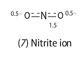### Periodicity and nomenclature

Valency was the original basis of the Periodic Table. Mendeleev found that, when elements are arranged in order of increasing atomic mass, a periodic repetition of valency is obtained.7 The only exceptions are where atomic mass does not parallel atomic number, and elements are missing. Valency therefore provides teachers with a simple way of introducing the Periodic Table. A major problem with using atomic structure is that students have to take so much on trust.

Chemists use valency in nomenclature. For Main Group atoms that exhibit variable valency, they choose a standard value and cite non-standard ones, using the symbol λv

### Electronic theory

The concept of valency played a part in the development of electronic theories of bonding. Lewis arrived at his structures of atoms and molecules by seeking to explain the valencies of the elements.10 The concept therefore leads students into his ideas,11 and into more advanced theories of bonding. I would encourage teachers to make use of it.

Dr Peter Nelson is a retired lecturer in the department of chemistry at the University of Hull, Cottingham Road, Hull HU6 7RX.